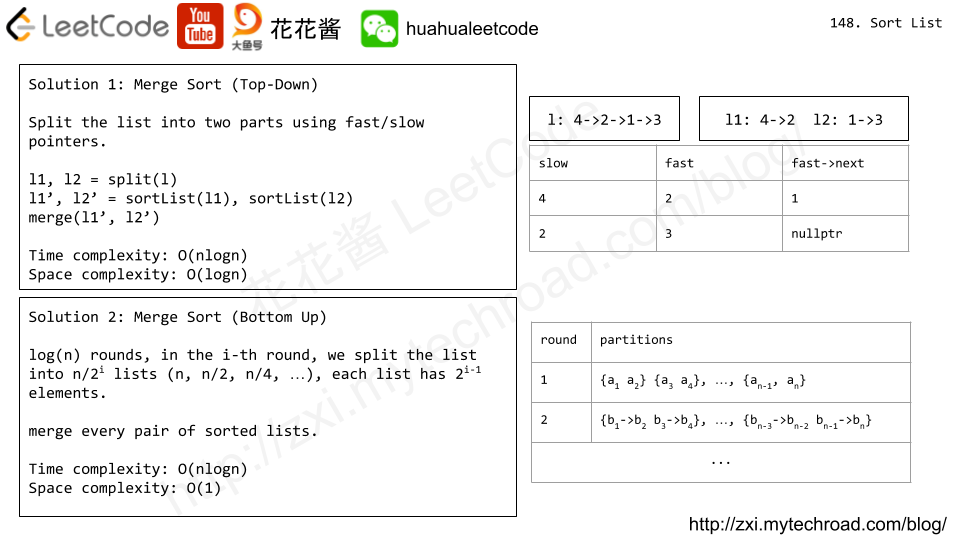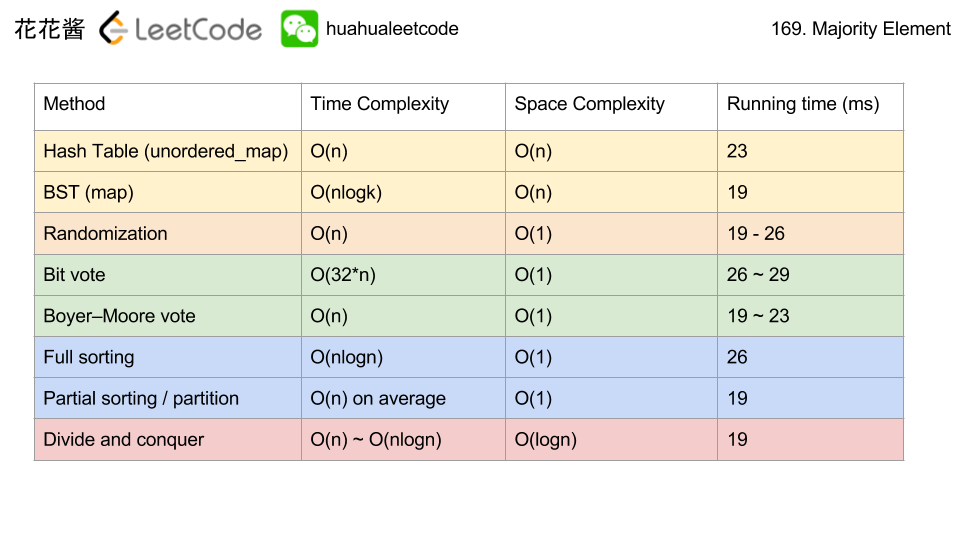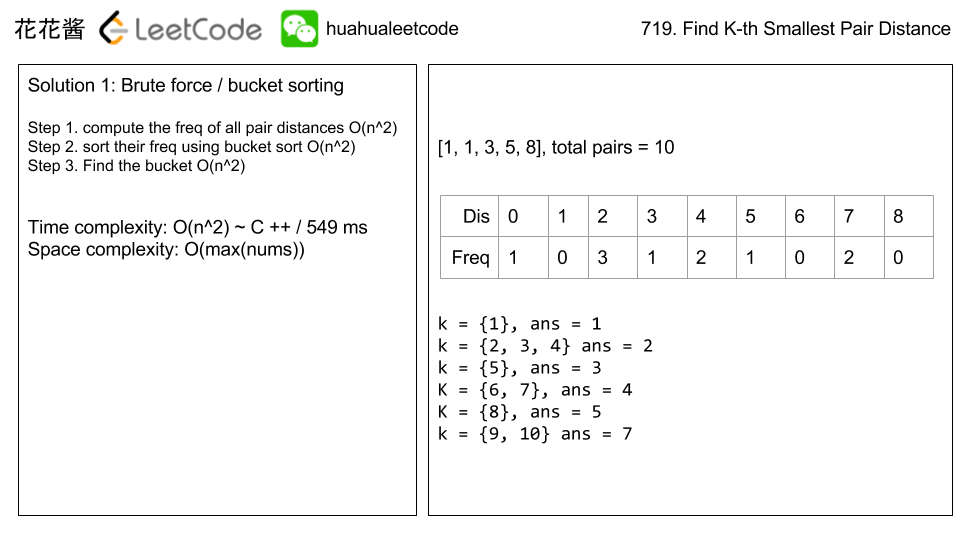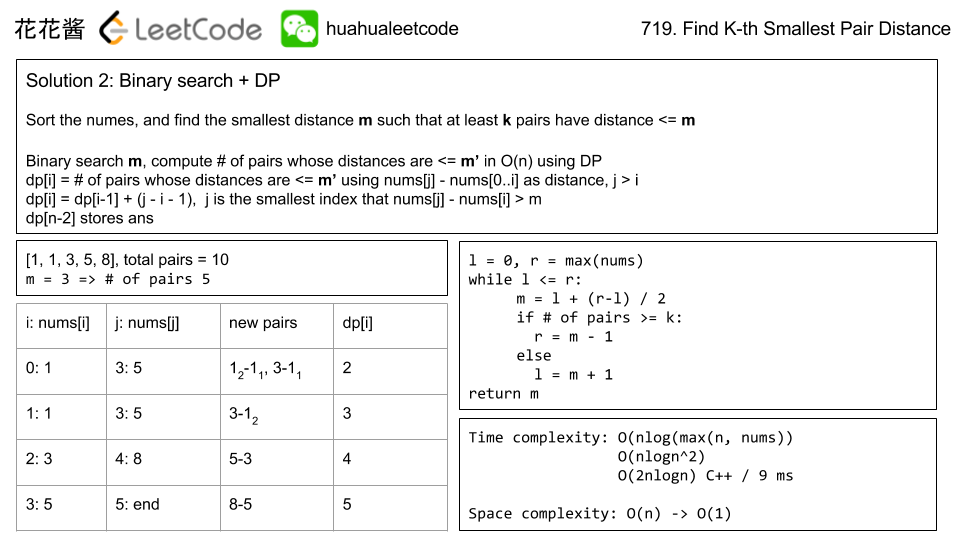# Posts published in “Divide and conquer”

(This problem is an interactive problem.)

On the sea represented by a cartesian plane, each ship is located at an integer point, and each integer point may contain at most 1 ship.

You have a function Sea.hasShips(topRight, bottomLeft) which takes two points as arguments and returns true if and only if there is at least one ship in the rectangle represented by the two points, including on the boundary.

Given two points, which are the top right and bottom left corners of a rectangle, return the number of ships present in that rectangle.  It is guaranteed that there are at most 10 ships in that rectangle.

Submissions making more than 400 calls to hasShips will be judged Wrong Answer.  Also, any solutions that attempt to circumvent the judge will result in disqualification.

Example :

Input:
ships = [[1,1],[2,2],[3,3],[5,5]], topRight = [4,4], bottomLeft = [0,0]
Output: 3
Explanation: From [0,0] to [4,4] we can count 3 ships within the range.


Constraints:

• On the input ships is only given to initialize the map internally. You must solve this problem “blindfolded”. In other words, you must find the answer using the given hasShips API, without knowing the ships position.
• 0 <= bottomLeft <= topRight <= 1000
• 0 <= bottomLeft <= topRight <= 1000

## Solution: Divide and Conquer

If the current rectangle contains ships, subdivide it into 4 smaller ones until
1) no ships contained
2) the current rectangle is a single point (e.g. topRight == bottomRight)

Time complexity: O(logn)
Space complexity: O(logn)

# Problem

Sort a linked list in O(n log n) time using constant space complexity.

Example 1:

Input: 4->2->1->3
Output: 1->2->3->4


Example 2:

Input: -1->5->3->4->0
Output: -1->0->3->4->5# Solution: Merge Sort

Top-down (recursion)

Time complexity: O(nlogn)

Space complexity: O(logn)

## Python3

bottom up

Time complexity: O(nlogn)

Space complexity: O(1)

# Problem

We have some permutation A of [0, 1, ..., N - 1], where N is the length of A.

The number of (global) inversions is the number of i < j with 0 <= i < j < N and A[i] > A[j].

The number of local inversions is the number of i with 0 <= i < N and A[i] > A[i+1].

Return true if and only if the number of global inversions is equal to the number of local inversions.

Example 1:

Input: A = [1,0,2]
Output: true
Explanation: There is 1 global inversion, and 1 local inversion.


Example 2:

Input: A = [1,2,0]
Output: false
Explanation: There are 2 global inversions, and 1 local inversion.


Note:

• A will be a permutation of [0, 1, ..., A.length - 1].
• A will have length in range [1, 5000].
• The time limit for this problem has been reduced.

# Solution1: Brute Force (TLE)

Time complexity: O(n^2)

Space complexity: O(1)

C++

# Solution2: MergeSort

Time complexity: O(nlogn)

Space complexity: O(n)

C#

# Solution3: Input Property

Input is a permutation of [0, 1, …, N – 1]

Time Complexity: O(n)

Space Complexity: O(1)

Problem:

Given an array of size n, find the majority element. The majority element is the element that appears more than ⌊ n/2 ⌋ times.

You may assume that the array is non-empty and the majority element always exist in the array.

Ideas:Solution 1:

Hash table O(n) / O(n)

Solution 2:

BST O(nlogk) / O(n)

Solution 3:

Randomization O(n) / O(1)

Solution 4:

Bit voting O(n) / O(1)

Solution 5:

Moore Voting O(n) / O(1)

Solution 6:

Full sorting O(nlogn) / O(1)

Solution 7:

Partial sorting O(n) / O(1)

Solution 8:

Divide and conquer O(nlogn) / O(logn)

Divide and conquer O(nlogn) / O(logn)

Problem:

Given an integer array, return the k-th smallest distance among all the pairs. The distance of a pair (A, B) is defined as the absolute difference between A and B.

Example 1:

Note:

1. 2 <= len(nums) <= 10000.
2. 0 <= nums[i] < 1000000.
3. 1 <= k <= len(nums) * (len(nums) - 1) / 2.

Idea

Bucket sort

Binary search / dpSolution

C++ / binary search

C++ / bucket sort w/ vector O(n^2)

Related Problems:

Mission News Theme by Compete Themes.